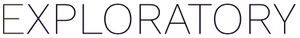# Averaging values by row

#1

Hi -

First time posting here…I have a pretty simple question. How would I take an average of the values by row — either across all columns or a specific subset of columns?

Thanks,
Seth

#2

There are several ways to take the average of all the column (each column) values for each row. This is probably not supported by Exploratory’s UI, so you’ll need to write a simple script to do it.

Here are two ways to do it. One is using `rowMeans()` and the other is using `appply()`.

First, if you want to calculate the average of all columns by rows, you can use one of the following methods.

``````rowMeans(.)

or

apply(., 1, mean)
``````

If you want to calculate the average per row over the selected columns, you can do it in one of the following ways. Besides `starts_with`, other useful functions for column extraction are provided in R. Refer to the following URL and use the functions you need.

``````rowMeans(select(., starts_with("int")))

or

apply(select(., starts_with("int")), 1, mean))
``````

I hope this helps.

#3

Unfortunately when I try to do that, Exploratory throws up an error code: [ Error : object ‘.’ not found ]

See screenshots:

Why is it doing that?

#4

Thanks for the screenshot. It helped me understand the current situation.

Please use “Create Caluclation(mutate)” instead of “R Script DataFrame” to write the column name and R script. Take a look at the image.